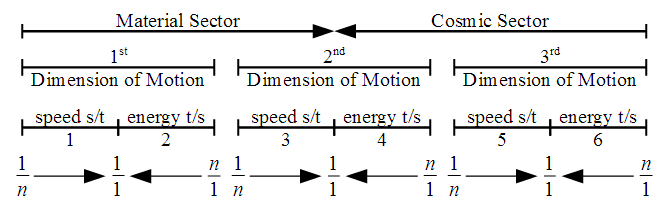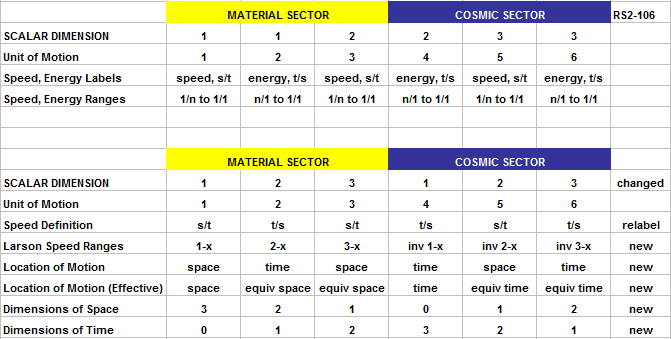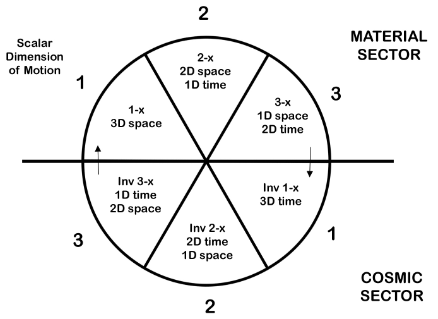## Scalar Dimension of Motion Diagrams

This forum is dedicated to the student just starting out with the concepts of the Reciprocal System, or RS2. Questions and clarifications for the RS/RS2 concepts go here; please place new ideas and commentary in the appropriate RS2 fora.
Philip
Posts: 7
Joined: Thu Dec 01, 2016 9:44 pm

### Scalar Dimension of Motion Diagrams

The first diagram was extracted from RS2-106.The second diagram (top portion) contains the same information as the preceding, but in my format. The second diagram (bottom portion) presents additional information which needs to be verified. Significantly, it updates the scalar dimension where motion occurs. I don’t know if this is correct, but I know that the scalar dimension associations shown in the original RS2-106 diagram are in doubt due to the work presented in RS2-109.The third diagram uses a circular format to more clearly indicate the flow of motion into an adjacent area. By redefining the scalar dimension where the motion occurs, the three scalar dimensions are all similar in that they link together (within a scalar dimension) the matching Larson speed ranges (i.e. 1-x with inv 1-x). Also, with this definition, the corresponding ranges (e.g. 1-x and inv 1-x) are opposed in the diagram.bperet
Posts: 1501
Joined: Thu Jul 22, 2004 1:43 am
Location: 7.5.3.84.70.24.606
Contact:

### Re: Scalar Dimension of Motion Diagrams

Larson has a number of logical inconsistencies in his work, and this is one of them. He states that the scalar dimensions are independent, which means they all must behave exactly the same. In the top diagram, it can be seen that the 2nd dimension does not, straddling the sector boundary.

RS2 includes the angular (yin) velocity component that treats all the speed ranges as orthogonal rotations, which you have actually diagrammed in the last figure as a 3D, quaternion rotation.

1-x: 1 → i
2-x: i → i.j
3-x: i.j → -1 (i.j.k)

What this also demonstrates is that the outward progression at unit speed is the start point, and inward gravitation at unit speed is the end point, per sector. Nehru discusses this in his paper on "Non-locality in the Reciprocal System". Might want to take a look at it, particularly Figure 4.

Note that 1-dimensional, electric rotations are limited to the 1-x speed range (a complex quantity), and because you cannot rotate in the same plane twice, i.i = -1 is not possible--hence, electrons and positrons cannot gravitate.
Last edited by bperet on Fri Dec 09, 2016 7:46 pm, edited 1 time in total.
Reason: Added PDF for Nehru's nonlocality paper
Every dogma has its day...